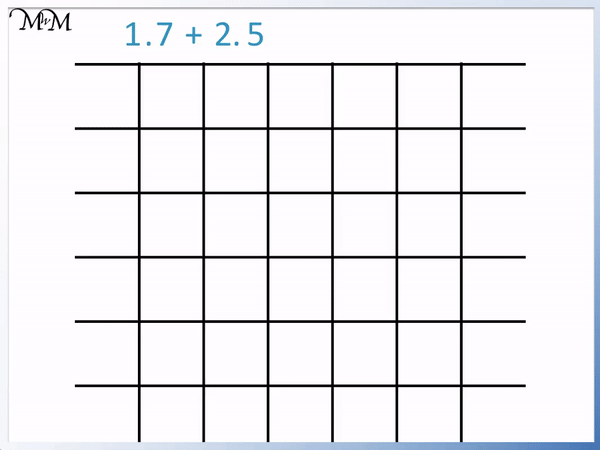• First line up the decimal points of each number.
• Line up the digits of the numbers that are in the same place value columns.
• Add the digits in each column from right to left.
• If the answer to this addition contains 2-digits, then carry the tens digit over to the next column.
• 7 + 5 = 12 and so we write the 2 below and carry the 1 over.
• We now add the digits in the next column, including the 1 we carried.
• 1 + 2 + the 1 we carried = 4.
• 1.7 + 2.5 = 4.2.

Line up the decimal points and the digits in each place value column.

Add each digit to the digit above.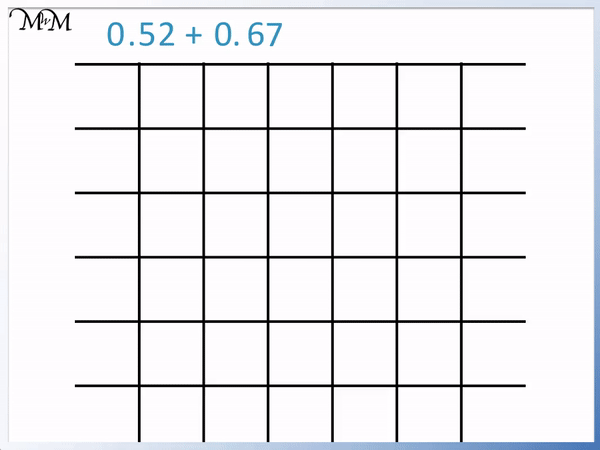• We line the decimal points up and then line up the digits of each number.
• We add the digits from the right to left.
• 2 + 7 = 9, so we write a 9 below.
• 5 + 6 = 11 and so, we write a 1 below and carry the 1 ten over.
• In the next column to the left of the decimal point we have 0 + 0 + the 1 we carried = 1.
• 0.52 + 0. 67 = 1.19.Supporting Lessons#### Column Addition of Decimals: Interactive Questions

To add decimals first line up the decimal points of each number and then line up the digits in each place value column. Add the digits separately from right to left.

Write the answers to each addition below the digits. Only write one digit in each place value column. If the answer is a two digit number, carry the ten over to add to the digits in the next column along.

We will consider the example of adding the decimal numbers 1.7 + 2.5.

The first step is to write one decimal above the other. It is important to first line up the decimal points of the numbers.

Then line up the digits in each place value column.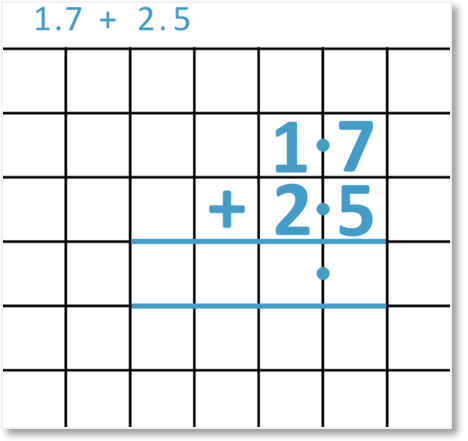Next, we add the digits from right to left.

Adding the digits in the tenths column, we have 7 + 5 = 12.

We only write 1 digit in each place value column. This means that we write the ‘2’ of ’12’ below and carry the ‘1’ into the next column on the left.

Write the carried digit below the answer lines.We now add the digits in the ones column. We have 1 + 2 + the 1 we carried earlier.

1 + 2 + 1 = 41.7 + 2.5 = 4.2In this next example we add the decimals 0.52 + 0.67.

The first step when adding two decimal numbers is to line up the decimal points.

We then line up the digits in the same place value columns.

We line up the 2 and the 7 in the hundredths column, the 5 and the 6 in the tenths column and the zeros in the ones column.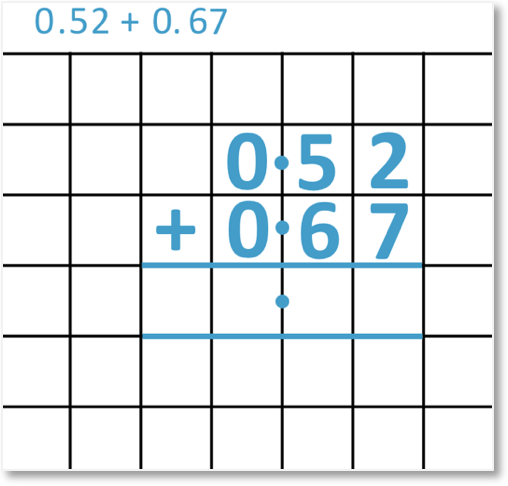We start by adding the digits from right to left, starting in the hundredths column.

2 + 7 = 9 and so, we write a 9 in the answer lines below.We now add the digits in the tenths column.

5 + 6 = 11 and so, we write a 1 below and carry the other 1 digit into the next column to the left.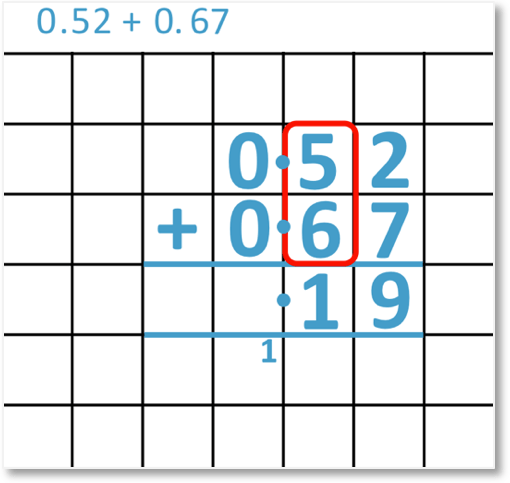We finally add the digits in the ones column. We have 0 + 0 + the 1 we carried. 0 + 0 + 1 = 1. We write this 1 in the answer space.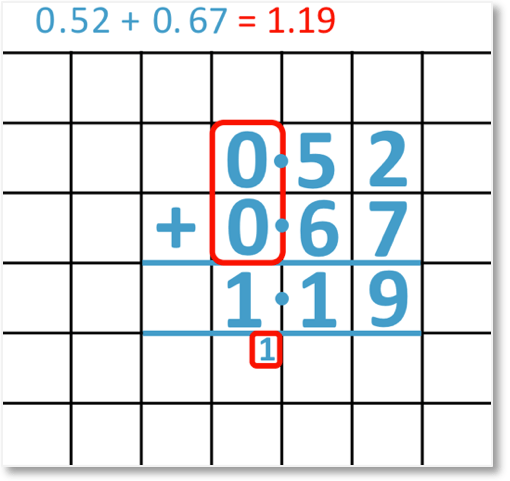## Adding Decimals with Different Place Values

To add decimals with different place values, line up the decimal points. Then line up the digits from left to right. Write zeros on the end of decimal numbers that have fewer digits than other numbers until the numbers have the same number of digits.

Then add the digits in each place value column separately, working from right to left.

For example, we will add the two decimals 3.4 and 1.58.

The decimal number 3.4 has just 2 digits whereas the decimal number 1.58 has 3 digits.

We first write the two numbers, lining up the decimal points and then the digits from left to right.

We put a 0 digit on the end of 3.4 in the hundredths column to make 3.40.The two decimal numbers now have the same number of place value columns.

We add the digits from right to left, starting in the hundredths column.

0 + 8 = 84 + 5 = 93 + 1 = 43.4 + 1.58 = 4.98

Here is another example of adding decimals with different place values. We have 5.07 + 7.4.

5.07 has 3 digits with the last digit in the hundredths column.

7.4 has 2 digits with the last digit in the tenths column.

we add a zero digit after 7.4 to make 7.40.Adding the digits in the hundredths column, 7 + 0 = 7.

Adding the digits in the tenths column, 0 + 4 = 4.

Adding the digits in the ones column, 5 + 7 = 12.

5.07 + 7.4 = 12.47Now try our lesson on Column Subtraction of 2-Digit Numbers where we learn how to use the column subtraction method.